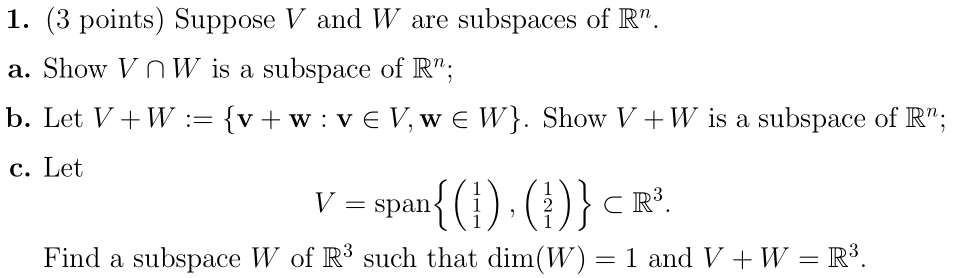Home / Answered Questions / Other / 1-3-points-suppose-v-and-w-are-subspaces-of-r-a-show-v-nw-is-a-subspace-of-r-b-let-v-w-v-w-vev-we-w--aw864

# (Solved): 1. (3 Points) Suppose V And W Are Subspaces Of Râ€. A. Show V NW Is A Subspace Of R"; B. Let V+W :=...1. (3 points) Suppose V and W are subspaces of Râ€. a. Show V NW is a subspace of R"; b. Let V+W := {v+w:VEV, WE W}. Show V+W is a subspace of R"; c. Let V = span{(1) (+)} CR%. Find a subspace W of R3 such that dim(W) = 1 and V + W = R3.

We have an Answer from Expert